# Dijkstra Algorithm - Finding Shortest Path

Graph

In this tutorial we will learn to find shortest path between two vertices of a graph using Dijkstra's Algorithm.

## Graph

Consider the following graph.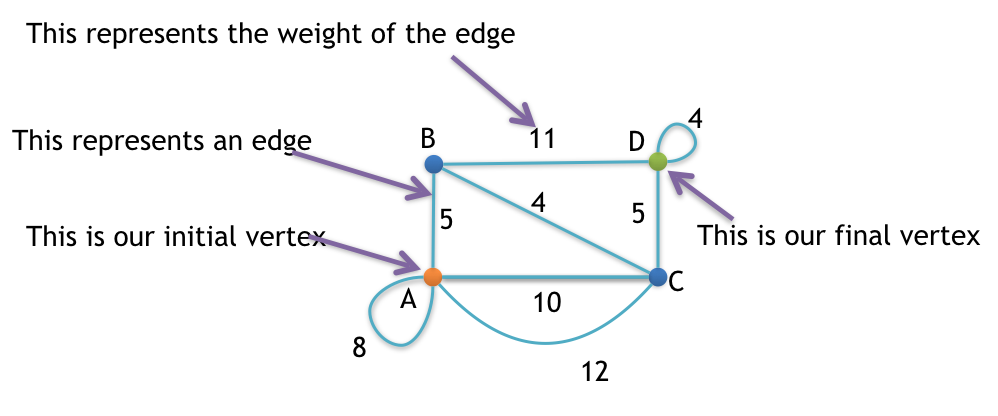## Steps

### Step 1: Remove all loops

Any edge that starts and ends at the same vertex is a loop.

Loops are marked in the image given below.### Step 2: Remove all parallel edges between two vertex except the one with least weight

In this graph, vertex A and C are connected by two parallel edges having weight 10 and 12 respectively. So, we will remove 12 and keep 10.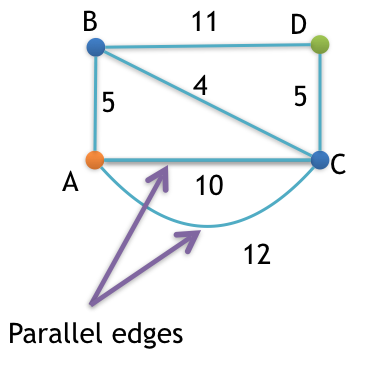We are now ready to find the shortest path from  vertex A to vertex D.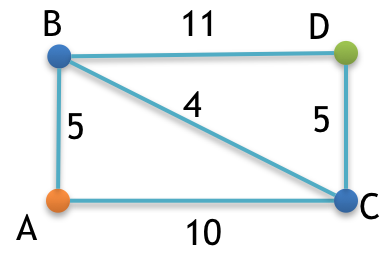### Step 3: Create shortest path table

As our graph has 4 vertices, so our table will have 4 columns.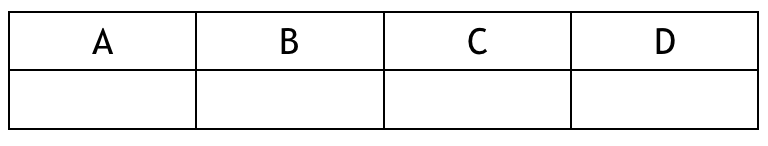Note! Column name is same as the name of the vertex.

After solving this we will have the following result. (See the above video for the steps)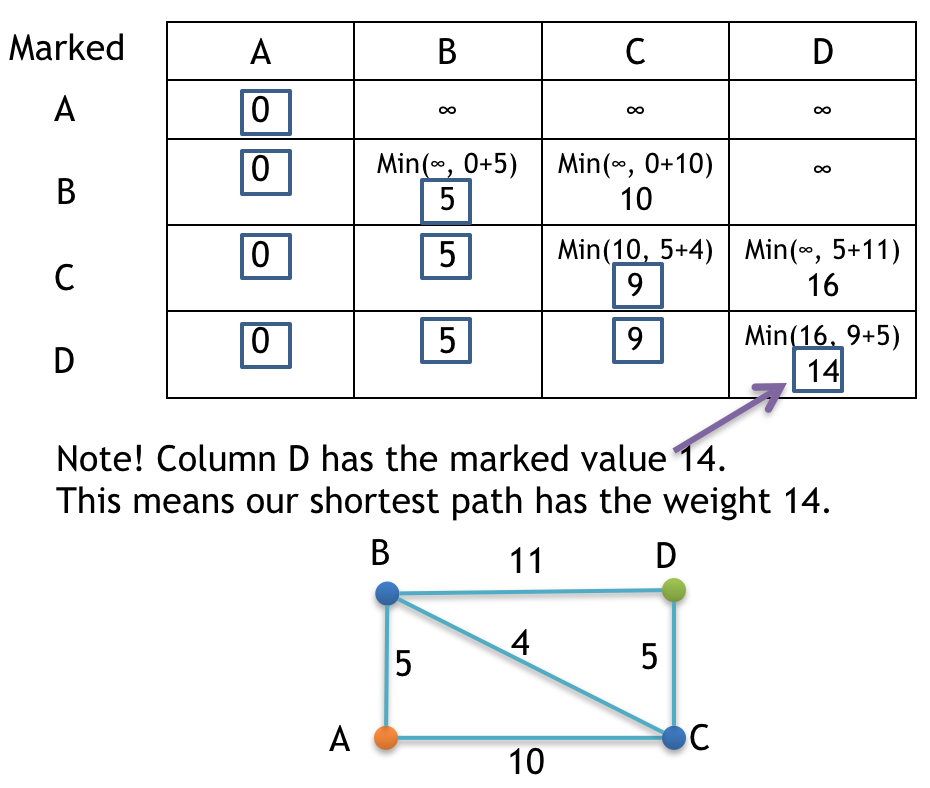## Result

Following is the required shortest path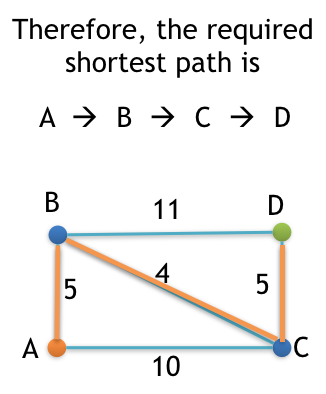## Code

Note! In the given code we are representing Vertices using decimal numbers.
So,
vertex A is denoted by digit 0.
vertex B is denoted by digit 1.
vertex C is denoted by digit 2.
vertex D is denoted by digit 3.

``````/**
* file: dijkstra.c
* author: yusuf shakeel
* date: 2014-03-03
*
* description: find shortest path betweem two vertices
*
* vertices are represented using numbers.
* vertex A becomes 0
* vertex B becomes 1
* and so on...
*/

#include <stdio.h>
#include <stdlib.h>

/**
* contant to represent infinity
* it is assumed that no edge in the vertex will have weight equal
* to this value.
*/
#define INF 9999

/**
* total number of vertices in the graph
*/
#define V 4

/**
* this function will return the minimum value
*/
int findMin(int x, int y) {
if (x < y) {
return x;
}
return y;
}

/**
* this function will check if the vertex is marked
*/
int isMarked(int v, int markedVertices[], int markedVerticesIdx) {

int i = 0;
for (i = 0; i < markedVerticesIdx; i++) {
if (v == markedVertices[i]) {
return 1;
}
}

return 0;
}

/**
* this function will find shortest path between source and destination vertex
*/
void dijkstra(int graph[V][V], int src, int dest) {

//variables
int i, r, c,
tmpC,
min,
currVertex,
edgeWt = 0,
destValue = 0,
markedValue = 0,
wtTableR = 0,
markedVerticesIdx = 0;

/**
* array containing vertices in the shortest path
*/
int shortestPathVertices[V] = {-1};
int shortestPathVerticesIdx = 0;

/**
* this table will hold the weight
*/
int weightTable[V][V];
for (r = 0; r < V; r++) {
for (c = 0; c < V; c++) {
weightTable[r][c] = INF;
}
}
weightTable[wtTableR++][src] = 0;

/**
* vertices that are marked
*/
int markedVertices[V] = {-1};
markedVertices[markedVerticesIdx++] = src;
currVertex = src;

/**
* find shortest path
*/
while(currVertex != dest) {

/**
* copy marked values to the next row of weightTable
*/
for (i = 0; i < markedVerticesIdx; i++) {
c = markedVertices[i];
weightTable[wtTableR][c] = weightTable[wtTableR - 1][c];
}

/**
* find the weight from the current vertex to all the other
* vertices that are directly connected and not yet marked
*/
for (c = 0; c < V; c++) {

if (c != currVertex && !isMarked(c, markedVertices, markedVerticesIdx)) {

edgeWt = graph[currVertex][c];
destValue = weightTable[wtTableR - 1][c];
markedValue = weightTable[wtTableR][currVertex];

min = findMin(destValue, markedValue + edgeWt);

weightTable[wtTableR][c] = min;

}

}

/**
* find minimum unmarked vertices in current row
*/
min = INF;
for (c = 0; c < V; c++) {

if (!isMarked(c, markedVertices, markedVerticesIdx)) {
if (weightTable[wtTableR][c] < min) {
min = weightTable[wtTableR][c];
tmpC = c;
}
}

}

/**
* found a new vertex for marking
*/
markedVertices[markedVerticesIdx++] = tmpC;
currVertex = tmpC;

/**
* variables update
*/
wtTableR++;

}

/**
* compute shortest path vertices
*/
c = dest;
shortestPathVertices[shortestPathVerticesIdx++] = c;
markedValue = weightTable[wtTableR - 1][dest];
for (r = wtTableR - 2; r >= 0; r--) {

if (weightTable[r][c] != markedValue) {
c = markedVertices[r];
markedValue = weightTable[r][c];
shortestPathVertices[shortestPathVerticesIdx++] = c;
}

}

/**
* display the weight and shortest path
*/
printf("Shortest Path between %d and %d\n", src, dest);
for (i = shortestPathVerticesIdx-1; i >= 0; i--) {
printf("%d", shortestPathVertices[i]);
if (i > 0) {
printf(" --> ");
}
}
printf("\n");
printf("Weight of the path: %d\n", weightTable[wtTableR-1][dest]);

}

int main(void) {

/**
* 2d array which holds the weight of the edges
*/
int graph[V][V] = {
{0, 5, 10, INF},
{5, 0, 4, 11},
{10, 4, 0, 5},
{INF, 11, 5, 0}
};

/**
* source and destination vertices
*/
int src = 0;
int dest = 3;

/**
* find shortest path
*/
dijkstra(graph, src, dest);

return 0;
}
``````

### Output

``````Shortest Path between 0 and 3
0 --> 1 --> 2 --> 3
Weight of the path: 14
``````